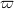﻿

### On dense subspaces of Cp(X)

#### Abstract

For a Tychonoff space X, we denote by Cp(X) the space of all real-valued continuous functions on X with the topology of pointwise convergence. We show the following:(1) if ω1 is a caliber for every dense subspace of Cp(X), then Cp X) is (ω1, ω1)-narrow; (2) if every dense subspace of Cp(X) is compact-dense in Cp(X), then every non-trivial countable$\varpi$-cover of open sets of X contains a γ-cover. The first result gives the positive answer to Problem 4.4 in , and the second one is a partial answer to Problem 4.3 in .

DOI Code: 10.1285/i15900932v27supn1p41

Keywords: Function space; Dense subspace; Caliber; (k, k)-compact; (k, k)-narrow; Linearly Lindelöf; ω-cover; γ-cover; Fréchet

Classification: 54C35; 54D20

Full Text: PDF